# Clarity

View Only

## Arithmetic with decimals/floats?#### L.Elias09-03-2014 01:01 PM#### Gurjeet.S09-04-2014 05:18 AM#### Georgy09-04-2014 05:49 AM#### L.Elias09-04-2014 09:22 AM#### Eugene Greiff09-04-2014 11:04 AM#### Georgy09-05-2014 05:42 AM#### Eugene Greiff09-05-2014 07:51 PM#### SagarSarkar09-27-2016 06:42 PM#### Eugene Greiff09-28-2016 12:57 AM#### SagarSarkar09-28-2016 09:50 AM#### Eugene Greiff09-28-2016 11:51 AM#### SagarSarkar09-28-2016 03:37 PM#### Eugene Greiff09-05-2014 11:08 PM#### Georgy09-08-2014 12:41 AM#### L.Elias09-15-2014 03:53 PM#### Anon Anon09-04-2014 11:16 AM• #### 1.  Arithmetic with decimals/floats?

Posted 09-03-2014 01:01 PM

I'm sure I'm just missing something extraordinarily obvious here but I can't get this to work. I have a GEL script that is querying the DB for some values to do some calculations. The data is stored in Clarity as a Number with 2 decimal places. It pulls and displays the numbers as I would expect, but it seems to be treating them as whole integers for the math

```<gel:log level="INFO" category="LOOP" var="include_log">Value \${t_weight} + \${t_value} = \${t_weight+t_value}</gel:log>
```

Leaves me with

[LOOP]Value 1.50 + 3 = 4

I also tried

```<core:expr value="\${t_weight + t_value}"/>
```

but got the same result. Multiplication does the same thing, giving stuff like 0.75 * 3 = 0

What am I missing here?

• #### 2.  Re: Arithmetic with decimals/floats?

Posted 09-04-2014 05:18 AM

Hi,

Possible BUG evaluating conditions

-Gurjeet

• #### 3.  Re: Arithmetic with decimals/floats?

Posted 09-04-2014 05:49 AM

Hi Elias,

Not sure what is different between your environment and mine. We are on v13.3 with Oracle and I ran the below sample GEL and got the correct output. x_decimal_one and x_decimal_two are decimals with 2 decimal places whereas x_integer_one is an integer with zero decimal places, created in the project object.

x_decimal_one=1.5

x_decimal_two=3

x_integer_one=3

<gel:script xmlns:core="jelly:core" xmlns:gel="jelly:com.niku.union.gel.GELTagLibrary" xmlns:sql="jelly:sql">

<gel:setDataSource dbId="Niku"/>

<sql:query var="errsql">

select x_decimal_one, x_decimal_two, x_integer_one from odf_ca_project where x_decimal_one is not null

</sql:query>

<core:set var="DEC_ONE">\${errsql.rows.x_decimal_one}</core:set>

<core:set var="DEC_TWO">\${errsql.rows.x_decimal_two}</core:set>

<core:set var="INT_ONE">\${errsql.rows.x_integer_one}</core:set>

<gel:log level="INFO" category="LOOP" var="include_log1">Value \${DEC_ONE} + \${DEC_TWO} = \${DEC_ONE+DEC_TWO}</gel:log>

<gel:log level="INFO" category="LOOP" var="include_log2">Value \${DEC_ONE} + \${INT_ONE} = \${DEC_ONE+INT_ONE}</gel:log>

</gel:script>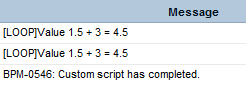Regards,

Georgy

• #### 4.  Re: Arithmetic with decimals/floats?

Posted 09-04-2014 09:22 AM

Hmm. I do seem to be having the same issue as in Gurjeet's post (that its casting/forcing the numbers to be integers) but I'm not doing any comparisons, just math. Not sure why Georgy's script seems to be working as its essentially the same sort of thing. I did forget to mention originally that we are on 13.0/MSSQL. I tried searching the support site and didn't see any knowledge docs related to this or any bugs. Was hoping it was just something easy I missed, looks like I should open a support ticket to see what they say.

• #### 5.  Re: Arithmetic with decimals/floats?

Posted 09-04-2014 11:04 AM

My suggestion is to figure out the underlying types (most likely strings) and parse them into the types you want.  If not strings, you will need to figure out the parse method.

I have had problems with this before.

```  <gel:log>\${t_value.getClass().getName()} </gel:log>
<gel:log>\${t_weight.getClass().getName()} </gel:log>

<core:invokeStatic className="java.lang.Float" method="parseFloat" var="floatValue">
<core:arg value="\${t_weight}" />
</core:invokeStatic>

<core:invokeStatic className="java.lang.Integer" method="parseInt" var="intValue">
<core:arg value="\${t_value}" />
</core:invokeStatic>

<gel:log level="INFO" category="LOOP" var="include_log">Value \${t_weight} + \${t_value} = \${floatValue+intValue}</gel:log>
```

V/r

Gene

• #### 6.  Re: Arithmetic with decimals/floats?

Posted 09-05-2014 05:42 AM

Hi Gene,

Tried this out of curiosity. Just wanted to see the parseint method converting a float number to integer in GEL.

I got the underlying type as  java.lang.String for all variables. But gives me the below error when t_value in the above script has value 1.5 in Clarity. Not sure if I am missing something here!

BPM-0704: An error occurred while executing custom script: org.apache.commons.jelly.JellyTagException: null:19:85: <core:invokeStatic> method parseInt threw exception: For input string: "1.5" at org.apache.commons.jelly.tags.core.InvokeStaticTag.doTag(InvokeStaticTag.java:148) at org.apache.commons.jelly.impl.TagScript.run(TagScript.java:247) at org.apache.commons.jelly.impl.ScriptBlock.run(ScriptBlock.java:95) at org.apache.commons.jelly.TagSupport.invokeBody(TagSupport.java:186) at com.niku.union.gel.tags.ScriptTag.doTag(ScriptTag.java:20) at org.apache.commons.jelly.impl.TagScript.run(TagScript.java:247) at com.niku.union.gel.GELScript.run(GELScript.java:48) at com.niku.union.gel.GELController.invoke(GELController.java:101) at com.niku.bpm.services.ExecuteCustomAction.run(ExecuteCustomAction.java:207) at java.util.concurrent.ThreadPoolExecutor.runWorker(ThreadPoolExecutor.java:1145) at java.util.concurrent.ThreadPoolExecutor\$Worker.run(ThreadPoolExecutor.java:615) at java.lang.Thread.run(Thread.java:724) Caused by: java.lang.NumberFormatException: For input string: "1.5" at java.lang.NumberFormatException.forInputString(NumberFormatException.java:65) at java.lang.Integer.parseInt(Integer.java:492) at java.lang.Integer.parseInt(Integer.java:527) at sun.reflect.NativeMethodAccessorImpl.invoke0(Native Method) at sun.reflect.NativeMethodAccessorImpl.invoke(NativeMethodAccessorImpl.java:57)

Regards,

Georgy

• #### 7.  Re: Arithmetic with decimals/floats?

Posted 09-05-2014 07:51 PM

You need to make sure that the string that you are parsing is really of the type you are looking for.

```<?xml version="1.0" encoding="UTF-8"?>
<gel:script
xmlns:core="jelly:core"
xmlns:gel="jelly:com.niku.union.gel.GELTagLibrary"
xmlns:xsd="http://www.w3.org/2001/XMLSchema"
xmlns:xsi="http://www.w3.org/2001/XMLSchema-instance">
<core:set var="t_weight" value="      1.5      "/>
<core:set var="t_value"  value="      3          "/>

<gel:log>\${t_value.getClass().getName()} </gel:log>
<gel:log>\${t_weight.getClass().getName()} </gel:log>

<core:invokeStatic className="java.lang.Float" method="parseFloat" var="floatValue">
<core:arg value="\${t_weight.trim()}" />
</core:invokeStatic>

<core:invokeStatic className="java.lang.Integer" method="parseInt" var="intValue">
<core:arg value="\${t_value.trim()}" />
</core:invokeStatic>

<gel:log level="INFO" category="LOOP" var="include_log">Value \${t_weight} + \${t_value} = \${floatValue+intValue}</gel:log>
</gel:script>
```

Here I have the string which contain a float and an integer.

I send the trimmed version of the string to the parse.  Here is the output.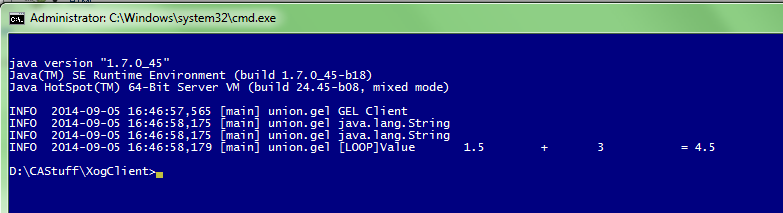V/r,

Gene

• #### 8.  Re: Arithmetic with decimals/floats?

Posted 09-27-2016 06:42 PM

Hi Gene,

I had a question on Rounding off float value in XOG. I have used parseFloat to convert a string type to Float and then I am trying to Round this off to the closest integer, just getting .intvalue() gives me 9 for 9.9 instead of 10.

Code:

<core:invokeStatic className="java.lang.Float" method="parseFloat" var="B">
<core:arg type="java.lang.String" value="\${B.toString().trim()}" />
</core:invokeStatic>

<core:invokeStatic className="java.lang.Math" method="round" var="C">
<core:arg value="\${B}" />
</core:invokeStatic>

This code gives me a no such method exception.

gcubed

• #### 9.  Re: Arithmetic with decimals/floats?

Posted 09-28-2016 12:57 AM

So core:set can be troublesome as you never know what type your var is.  For example, when I add 0.5 to my float values I end up with java.lang.Double instead of java.lang.Float.  If you try and add 0.5f to the float the parser just throws lines of exceptions!

<?xml version="1.0" encoding="UTF-8"?>
<gel:script xmlns:core="jelly:core"
xmlns:gel="jelly:com.niku.union.gel.GELTagLibrary"
xmlns:xsd="http://www.w3.org/2001/XMLSchema"
xmlns:xsi="http://www.w3.org/2001/XMLSchema-instance">

<core:set var="t_weight" value="      1.6       "/>
<core:set var="t_value"   value="      3.2          "/>

<gel:log>\${t_value.getClass().getName()} </gel:log>
<gel:log>\${t_weight.getClass().getName()} </gel:log>

<core:invokeStatic className="java.lang.Float" method="parseFloat" var="floatValue1">
<core:arg value="\${t_weight.trim()}" />
</core:invokeStatic>

<core:invokeStatic className="java.lang.Float" method="parseFloat" var="floatValue2">
<core:arg value="\${t_value.trim()}" />
</core:invokeStatic>

<core:set var="floatValue1"  value="\${floatValue1 + 0.5}" />
<gel:log>Round to nearest int = \${floatValue1.intValue()}</gel:log>

<core:set var="floatValue2"  value="\${floatValue2 + 0.5}" />
<gel:log>Round to nearest int = \${floatValue2.intValue()}</gel:log>

</gel:script>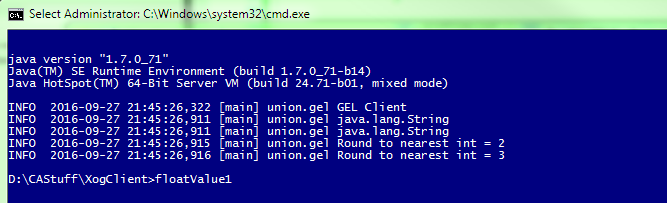V/r,

Gene

• #### 10.  Re: Arithmetic with decimals/floats?

Posted 09-28-2016 09:50 AM

Thanks gcubed.  I was cracking my head trying to use Math Library functions and solution was pretty straight forward I guess.

But in general is there any other tag that could help specify which type of variables are we using, I am doing a lot of calculations in my process it might be helpful down the line.

Can we use other JSTL Tags like the Format Tags from the "

• #### 11.  Re: Arithmetic with decimals/floats?

Posted 09-28-2016 11:51 AM

You can use any tag that is available in the lib directory.  Not all the Jelly tags are there but most of the basic one are.  If you are not in OnDemand you can build your own taglibs which would be available to Gel Scripts.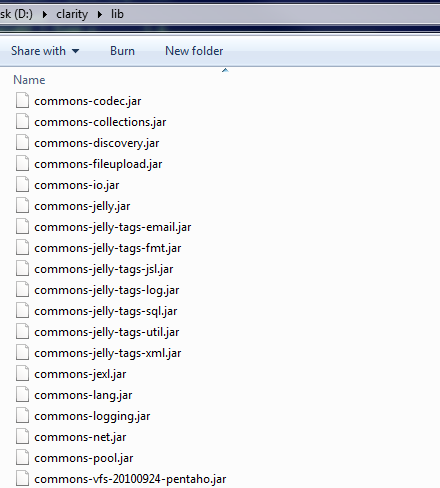So to get a var type:

``<?xml version="1.0" encoding="UTF-8"?><gel:script xmlns:core="jelly:core"xmlns:gel="jelly:com.niku.union.gel.GELTagLibrary"xmlns:xsd="http://www.w3.org/2001/XMLSchema"xmlns:xsi="http://www.w3.org/2001/XMLSchema-instance">     <core:set var="t_weight" value="      1.6       "/>     <core:invokeStatic className="java.lang.Float" method="parseFloat" var="floatValue1">           <core:arg value="\${t_weight.trim()}" />      </core:invokeStatic>          <gel:log>t_weight type = \${t_weight.getClass().getName()} </gel:log>     <gel:log>floatValue1 type = \${context.getVariable('floatValue1').getClass().getName()}</gel:log>     <!-- Dump the context -->     <gel:log />     <gel:log>Dump the context</gel:log>     <core:set var="entries" value="\${context.getVariables().entrySet().toArray()}" />     <core:forEach var="entry" items="\${entries}">          <core:if test="\${!entry.getKey().equalsIgnoreCase('systemScope')}" >          <gel:log> Key = \${entry.getKey()} | Type = \${entry.getValue()} </gel:log>          </core:if>     </core:forEach>     <core:set var="floatValue1"  value="\${floatValue1 + 0.5}" />     <gel:log>Round to nearest int = \${floatValue1.intValue()}</gel:log></gel:script>``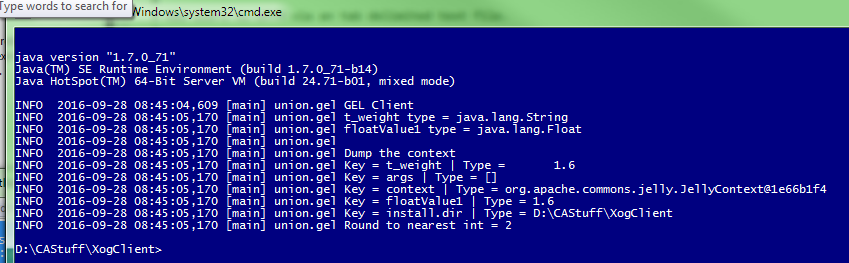V/r,Gene

• #### 12.  Re: Arithmetic with decimals/floats?

Posted 09-28-2016 03:37 PM

Thanks a lot. This was helpful.

• #### 13.  Re: Arithmetic with decimals/floats?

Posted 09-05-2014 11:08 PM

The other option is to convert each string to a float and then take the intValue of the float that you want an integer.

```<?xml version="1.0" encoding="UTF-8"?>
<gel:script
xmlns:core="jelly:core"
xmlns:gel="jelly:com.niku.union.gel.GELTagLibrary"
xmlns:xsd="http://www.w3.org/2001/XMLSchema"
xmlns:xsi="http://www.w3.org/2001/XMLSchema-instance">
<core:set var="t_weight" value="      1.5       "/>
<core:set var="t_value"   value="      3.2          "/>

<gel:log>\${t_value.getClass().getName()} </gel:log>
<gel:log>\${t_weight.getClass().getName()} </gel:log>

<core:invokeStatic className="java.lang.Float" method="parseFloat" var="floatValue1">
<core:arg value="\${t_weight.trim()}" />
</core:invokeStatic>

<core:invokeStatic className="java.lang.Float" method="parseFloat" var="floatValue2">
<core:arg value="\${t_value.trim()}" />
</core:invokeStatic>

<gel:log level="INFO" category="LOOP" var="include_log">Value \${t_weight} + \${t_value} != \${floatValue1+floatValue2.intValue()} because t_value int = \${floatValue2.intValue()}</gel:log>
</gel:script>
```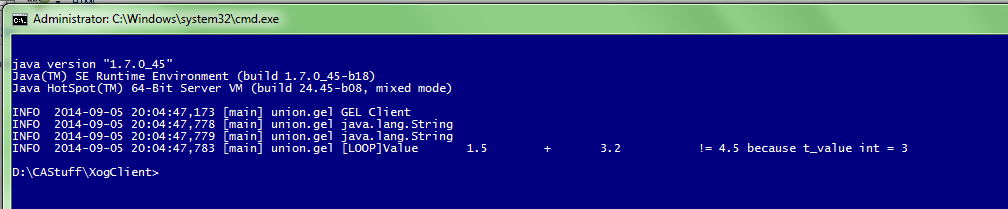V/r,

Gene

• #### 14.  Re: Arithmetic with decimals/floats?

Posted 09-08-2014 12:41 AM

Thanks a lot Gene for the detailed explanation

Regards,

Georgy

• #### 15.  Re: Arithmetic with decimals/floats?

Posted 09-15-2014 03:53 PM

Thanks for all the help Gene, sorry it took so long to get back to this.

```\${t_value.getClass().getName()}

```

Returned BigDecimal for me. Was able to cast to a double while setting

```<core:set var="t_value" value="\${row.get(var_column).doubleValue()}" />

```

That looked like it fixed it until I got to this combination

```0.8 * 7.0 = 5.6000000000000005

```

I tried with float instead of double, same result. Also set t_value to intValue since it will always be an int anyway, no change.

Edit: Scratch that, upon further review it looks like it wasn't ALWAYS being cast to a BigDecimal to begin with. By forcing that conversion, leaving them as BigDecimal, and using its multiply function, this appears to be working now:

```<core:new className="java.math.BigDecimal" var="t_value">
<core:arg type="java.lang.String" value="\${row.get(var_column).toString() }" />
</core:new>
<core:new className="java.math.BigDecimal" var="t_weight">
<core:arg type="java.lang.String" value="\${m_weights.get(gm_weights_mapping.get(var_column)).toString() }" />
</core:new>
<gel:log level="INFO" category="ALIGN" var="include_log">Value \${t_column} [\${t_weight_map}]: \${t_weight} * \${t_value} = \${t_value.multiply(t_weight)}</gel:log>
```

• #### 16.  Re: Arithmetic with decimals/floats?

Posted 09-04-2014 11:16 AM

You could just use a <sql:query> tag and put any math calculations inside it to get values that you want. You can call Stored procedures or functions to perform more complex operations.

Regards,

AK

• #### 17.  Re: Arithmetic with decimals/floats?

Posted 01-31-2017 03:29 PM

As Gene pointed out, the reason you will see behavior like this is because of the underlying types of each variable, what operators they support and what their toString() method does.

If you + (concatenation operator) a variable whose class doesn't support the operator it will toString it and then perform the concatenation.  If you are using two variables whose class does support the operator then the operation will be performed but you will still be at the mercy of what that class's toString method does afterwards unless you are formatting it explicitly the way you want.  In some cases (like with BigDecimal) it looks like it is cast to an int.  In general, only primitives support arithmetic operators.  To do arithmetic on objects (instances of classes) call their functions.

Also, the biggest reason I replied to this is because the classes that are used to represent different data types are database architecture dependent.  For example, you can have a different class for a date by running the same query on SQL Server and Oracle.  The same holds true for decimals and other data types.  They won't always be the same cross-platform.